# Generalized Linear Models

Let the outcome belong to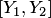. We say that Expert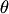's prediction at stepis denoted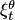and is equal to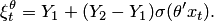Here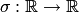is a fixed \emph{activation function}. We have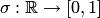in all the cases except linear regression (see below). If the range of the function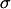is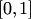, the experts necessarily give predictions from.

• Logistic activation function is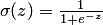.
• Probit activation function is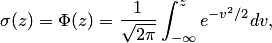where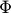is the cumulative distribution function of the normal distribution with zero mean and unit variance.

• Complementary log-log (comlog) activation function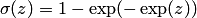.

Generalized Linear Models are often used for classification purposes.

### Bibliography

• McCullagh, P., Nelder, J. Generalized Linear Models. Chapman & Hall/CRC, second edn. 1989.